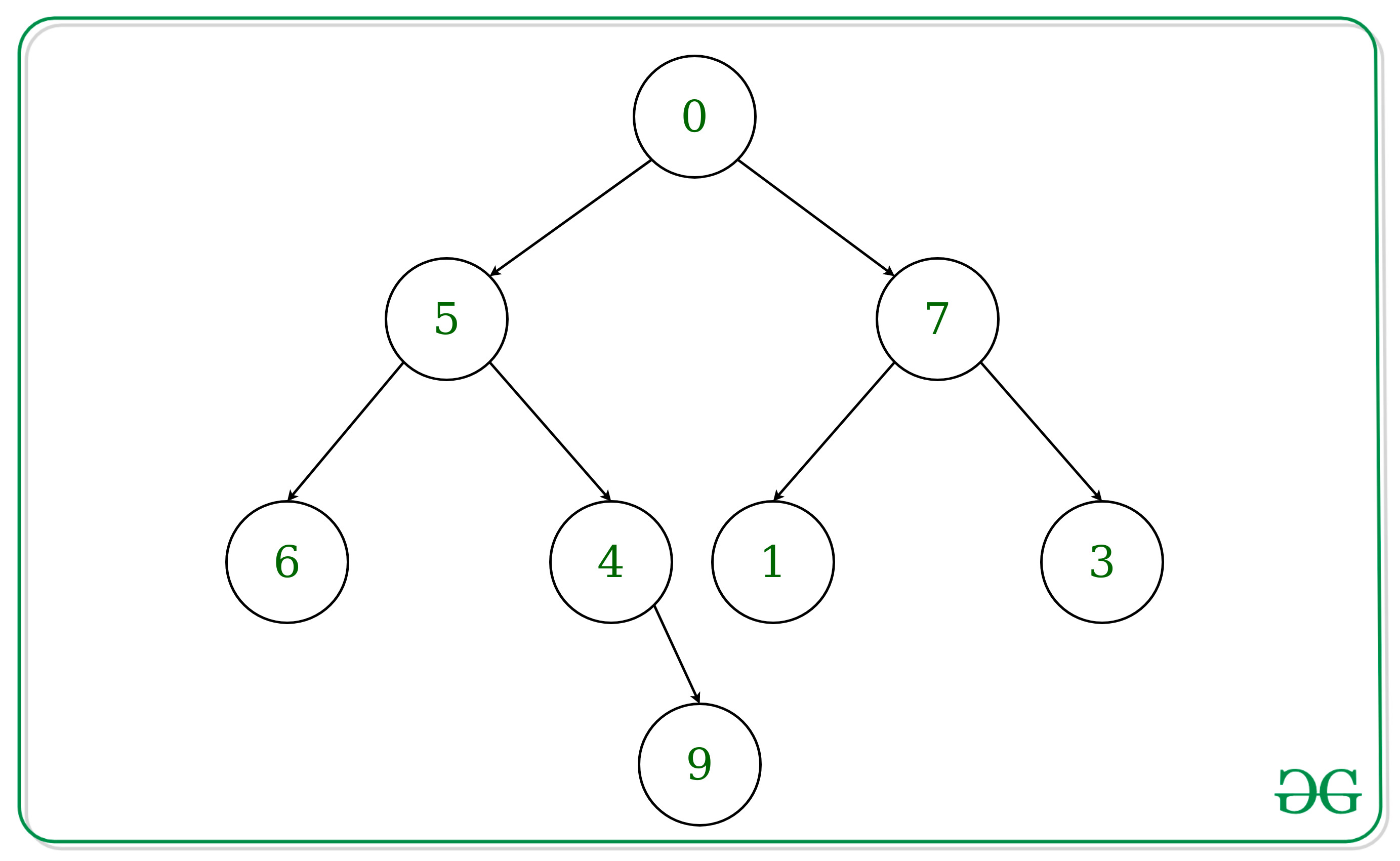Open in App
Not now

# Product of nodes at k-th level in a tree represented as string using Recursion

• Last Updated : 25 Sep, 2022

Prerequisite: Product of nodes at k-th level in a tree represented as string
Given an integer ‘K’ and a binary tree in string format. Every node of a tree has value in range from 0 to 9. We need to find product of elements at K-th level from the root. The root is at level 0.
Note: Tree is given in the form: (node value(left subtree)(right subtree))
Examples:

Input: Tree = “(0(5(6()())(4()(9()())))(7(1()())(3()())))”
k = 2
Output: 72
Explanation:
Its tree representation is shown belowElements at level k = 2 are 6, 4, 1, 3
product of these elements = 6 * 4 * 1 * 3 = 72

Input: Tree = “(8(3(2()())(6(5()())()))(5(10()())(7(13()())())))”
k = 3
Output: 15
Elements at level k = 3 are 5, 1 and 3
product of these elements = 5 * 1 * 3 = 15

Approach: The idea is to treat the string as a tree without actually creating one, and simply traverse the string recursively in Postorder Fashion and consider nodes that are at level k only.

Below is the implementation of the above approach:

## C++

 `// C++ implementation to find product``// of elements at k-th level` `#include ``using` `namespace` `std;` `// Recursive Function to find product``// of elements at k-th level``int` `productAtKthLevel(string tree,``            ``int` `k, ``int``& i, ``int` `level){` `    ``if` `(tree[i++] == ``'('``) {` `        ``// if subtree is null,``        ``// just like if root == NULL``        ``if` `(tree[i] == ``')'``)``            ``return` `1;` `        ``int` `product = 1;` `        ``// Consider only level k node``        ``// to be part of the product``        ``if` `(level == k)``            ``product = tree[i] - ``'0'``;` `        ``// Recur for Left Subtree``        ``int` `leftproduct = productAtKthLevel(``                    ``tree, k, ++i, level + 1);` `        ``// Recur for Right Subtree``        ``int` `rightproduct = productAtKthLevel(``                ``tree, k, ++i, level + 1);` `        ``// Taking care of ')' after``        ``// left and right subtree``        ``++i;``        ``return` `product * leftproduct *``                       ``rightproduct;``    ``}``}` `// Driver Code``int` `main()``{``    ``string tree = ``"(0(5(6()())(4()"``    ``"(9()())))(7(1()())(3()())))"``;``    ``int` `k = 2;``    ``int` `i = 0;` `    ``cout << productAtKthLevel(tree, k, i, 0);` `    ``return` `0;``}`

## Java

 `// Java implementation to find``// product of elements at k-th level` `class` `GFG {``    ``static` `int` `i;` `    ``// Recursive Function to find product``    ``// of elements at k-th level``    ``static` `int` `productAtKthLevel(``        ``String tree, ``int` `k, ``int` `level){` `        ``if` `(tree.charAt(i++) == ``'('``) {` `            ``// if subtree is null,``            ``// just like if root == null``            ``if` `(tree.charAt(i) == ``')'``)``                ``return` `1``;` `            ``int` `product = ``1``;` `            ``// Consider only level k node``            ``// to be part of the product``            ``if` `(level == k)``                ``product = tree.charAt(i) - ``'0'``;` `            ``// Recur for Left Subtree``            ``++i;``            ``int` `leftproduct = productAtKthLevel(``                            ``tree, k, level + ``1``);` `            ``// Recur for Right Subtree``            ``++i;``            ``int` `rightproduct = productAtKthLevel(``                            ``tree, k, level + ``1``);` `            ``// Taking care of ')' after``            ``// left and right subtree``            ``++i;``            ``return` `product * leftproduct``              ``* rightproduct;``        ``}``        ``return` `Integer.MIN_VALUE;``    ``}` `    ``// Driver Code``    ``public` `static` `void` `main(String[] args)``    ``{``        ``String tree = ``"(0(5(6()())(4()"``        ``+ ``"(9()())))(7(1()())(3()())))"``;``        ``int` `k = ``2``;``        ``i = ``0``;``        ``System.out.print(``            ``productAtKthLevel(tree, k, ``0``)``        ``);``    ``}``}`

## Python

 `# Python implementation to find product of``# digits of elements at k-th level` `# Recursive Function to find product``# of elements at k-th level``def` `productAtKthLevel(tree, k, i, level):``    ` `    ``if``(tree[i[``0``]]``=``=``'('``):``        ``i[``0``]``+``=` `1``        ``# if subtree is null,``        ``# just like if root == NULL``        ``if``(tree[i[``0``]] ``=``=` `')'``):``            ``return` `1`           `        ` `        ``product ``=` `1``        ``# Consider only level k node``        ``# to be part of the product``        ``if``(level ``=``=` `k):``            ``product ``=` `int``(tree[i[``0``]])``            ` `        ``# Recur for Left Subtree``        ``i[``0``]``+``=` `1``        ``leftproduct ``=` `productAtKthLevel(tree,``                            ``k, i, level ``+` `1``)``            ` `        ``# Recur for Right Subtree``        ``i[``0``]``+``=` `1``        ``rightproduct ``=` `productAtKthLevel(tree,``                            ``k, i, level ``+` `1``)``            ` `        ``# Taking care of ')' after left and right subtree``        ``i[``0``]``+``=` `1``        ``return` `product ``*` `leftproduct ``*` `rightproduct        ``    ` `# Driver Code``if` `__name__ ``=``=` `"__main__"``:``    ``tree ``=` `"(0(5(6()())(4()(9()())))(7(1()())(3()())))"``    ``k ``=` `2``    ``i ``=``[``0``]``    ``print``(productAtKthLevel(tree, k, i, ``0``))`

## C#

 `// C# implementation to find product``// of elements at k-th level` `using` `System;` `class` `GFG {``    ``static` `int` `i;` `    ``// Recursive Function to find product``    ``// of elements at k-th level``    ``static` `int` `productAtKthLevel(``        ``String tree, ``int` `k, ``int` `level){` `        ``if` `(tree[i++] == ``'('``) {` `            ``// if subtree is null,``            ``// just like if root == null``            ``if` `(tree[i] == ``')'``)``                ``return` `1;` `            ``int` `product = 1;` `            ``// Consider only level k node``            ``// to be part of the product``            ``if` `(level == k)``                ``product = tree[i] - ``'0'``;` `            ``// Recur for Left Subtree``            ``++i;``            ``int` `leftproduct = productAtKthLevel(``                            ``tree, k, level + 1);` `            ``// Recur for Right Subtree``            ``++i;``            ``int` `rightproduct =``            ``productAtKthLevel(tree, k, level + 1);` `            ``// Taking care of ')' after``            ``// left and right subtree``            ``++i;``            ``return` `product *``              ``leftproduct * rightproduct;``        ``}``        ``return` `int``.MinValue;``    ``}` `    ``// Driver Code``    ``public` `static` `void` `Main(String[] args)``    ``{``        ``String tree = ``"(0(5(6()())(4()"``        ``+``"(9()())))(7(1()())(3()())))"``;``        ``int` `k = 2;``        ``i = 0;``        ``Console.Write(productAtKthLevel(tree, k, 0));``    ``}``}`

## Javascript

 ``

Output:

`72`

Time Complexity: O(N)
Auxiliary space: O(N), for recursive stack space.

My Personal Notes arrow_drop_up